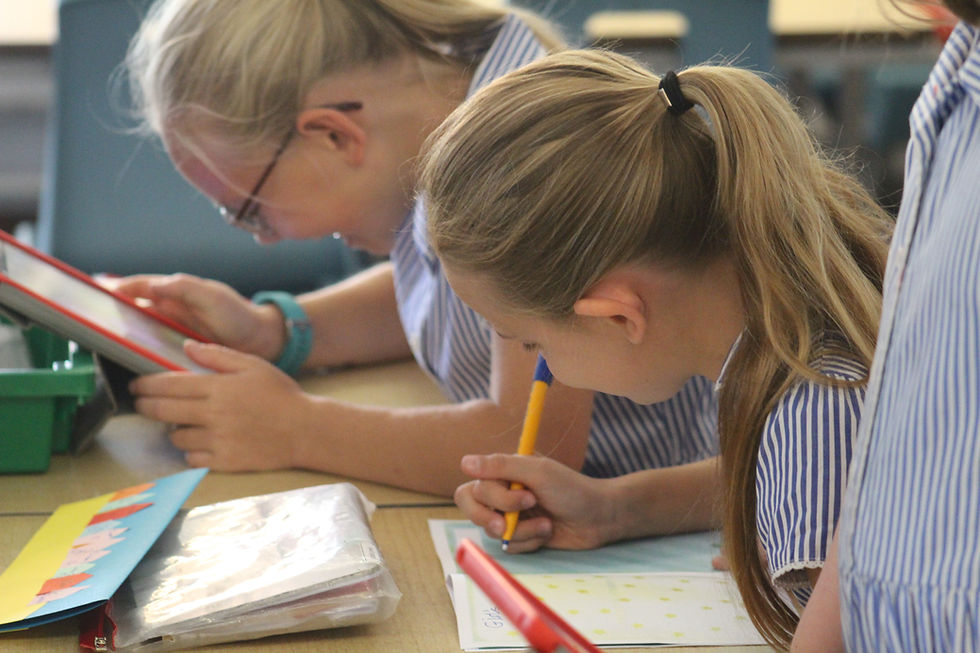top of page## ST KATHARINE'S LEARNING HUB

Search
• St Katharine's

# Year 3 Home Learning - Week 8

Year 3 Home Learning Overview
.pdf
Life Cycles Overview EY - Y3
.pdf
Years 3 and 4 RE
.pdf

White Rose Maths Lessons 1-4

Y3 Lesson 1 Add and subtract 2-digit and
Y3 ANS1 Add and subtract 2-digit and 3-d
Y3 Lesson 2 Add 2-digit and 3-digit numb
Y3 ANS2 Add 2-digit and 3-digit numbers
Y3 Lesson 3 Subtract 2-digit numbers fro
Y3 ANS3 Subtract 2-digit numbers from 3-
Y3 Lesson 4 Add two 3-digit numbers - no
Y3 ANS4 Add two 3-digit numbers - not cr